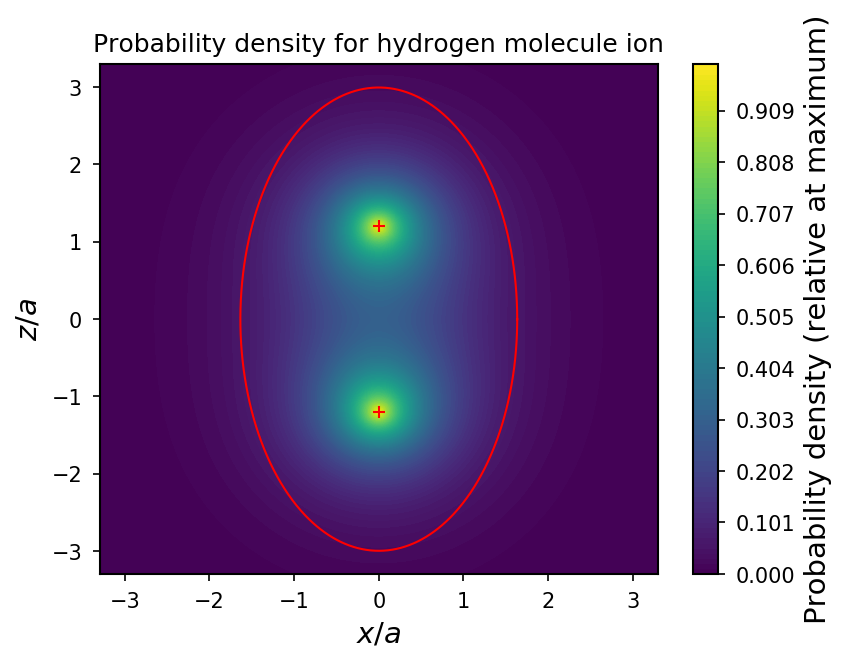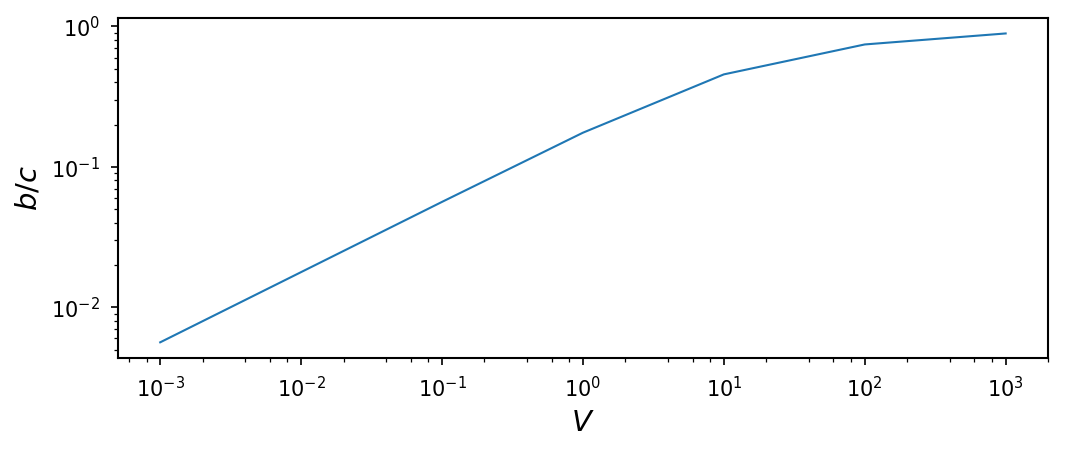# Determining the Shape of the Hydrogen Molecule Ion¶

## Examples - Quantum Mechanics¶

Last edited: January 19th 2019

The aim of this example is to determine the "shape of highest probability" for the hydrogen molecule ion. For a given volume, the task is to use Monte Carlo integration to find the ratio between the semi-principal axes of an ellipsoid, such that the probability of finding the electron inside the ellipsoid is maximised. If you are unfamiliar with Monte Carlo integration, take a look at the short introduction given in this notebook.

We are interested in determining the shape of the hydrogen molecule ion, that is one electron bound to two protons. The Hamiltonian for this system is $$H = -\frac{\hbar^2}{2m}\nabla^2 - \frac{e^2}{4 \pi \epsilon_o}\left(\frac{1}{r_1}+\frac{1}{r_2}\right).$$ Using the 1s wave function $\psi_{100}$ for hydrogen as a basis, we suggest the trial function $$\psi = A[\psi_{100}(r_1)+\psi_{100}(r_2)].$$ The normalization constant is (see e.g. Griffiths p. 306 ) $$A = \sqrt{\frac{1}{2(1+I)}}$$ with $$I = \exp(-R/a)\left[1+\frac{R}{a}+\frac{1}{3}\left(\frac{R}{a}\right)^2\right],$$ where $R$ is the distance between the protons. As calculated on page 308 in Griffiths , the distance which minimizes the energy is $R=2.4a$, where $a= 0.529$ Å is the Bohr radius. However, in order to avoid rounding errors, we will set $a=1$ in the following.

We assume azimutal symmetry, i.e. no $\phi$-dependence, such that the semi-principal axes in two directions, say the $x$- and $y$-directions, are equal. Then the two protons are placed on the $z$-axis, e.g. at $\pm R/2 \hat{z}$. For a given volume of the ellipsoid, $$V_0 = \frac{4}{3}\pi(2a)^3,$$ we want to find the ratio between the semi-principal axes $b$ and $c$ of the ellipsoid which maximizes the probability of the electron to be found inside the ellipsoid.

We will also need that the surface of an ellipsoid is described by $$\frac{x^2}{b^2}+\frac{y^2}{b^2}+\frac{z^2}{c^2} = 1$$ and that the volume is given by $$V = \frac{4}{3}\pi b^2 c.$$

Now we're good to go!

In :
%matplotlib inline
from __future__ import division
import matplotlib.pyplot as plt
import numpy as np
import random
import matplotlib.collections as collections

# Set common figure parameters:
newparams = {'axes.labelsize': 14, 'axes.linewidth': 1, 'savefig.dpi': 200,
'lines.linewidth': 1.0, 'figure.figsize': (2, 2),
'ytick.labelsize': 10, 'xtick.labelsize': 10,
'legend.fontsize': 10, 'legend.frameon': False,
'legend.handlelength': 1.5, 'figure.dpi': 150}
plt.rcParams.update(newparams)


First of all we define a function psi(r1,r2,R) which returns the value of the wave funtion for given distances $r_1$ and $r_2$ to the two protons, and the distance $R$ between the protons.

In :
def psi(r1, r2, R):
"""Wavefunction for an electron in a potential from two protons.
Input:
r1     Distance to proton 1
r2     Distance to proton 2
R      Distance between protons"""
a = 1 # Bohr radius (set to one)
I = np.exp(-R/a)*(1+R/a+1/3*(R/a)**2)
A = np.sqrt(1/(2*(1+I)))
return A/np.sqrt(np.pi*a**3)*(np.exp(-r1/a)+np.exp(-r2/a))


Next we define the number of random points, $N$, and use the general prosedure described here to calculate the probability inside an ellipsoid with semi-principal axes $b$ and $c$. Since the volume of the ellipsoid should be equal to the volume $V_0$, $c$ is determined from a chosen value of $b$.

In :
N = 1.0e5 # Number of random numbers
a = 1     # Bohr radius (set to one)
R = 2.4*a # From Griffiths p. 308
i = 0
n = 0
V_0 = (4/3)*np.pi*(2*a)**3  # Given volume


In the following code, we find the value of $b$ which maximizes the probability. $2a$ is chosen as an upper bound, since it seems reasonable that the ellipsoid should be stretched in the $z$-direction, meaning $b<c$ and hence $b<2a$, which corresponds to a sphere with the given volume $V_0$.

In :
"""The following code calculates the value of b which maximizes the probability inside the ellipsoid.
This is however not a very efficient solution."""

b_min = 1*a  # Lower limit for b
b_max = 2*a  # Upper limit for b, corresponds to sphere
b_steps = 6  # Number of steps between b_min and b_max
b_ = np.linspace(b_min, b_max, b_steps)  # Array of b-values, the lenght of semi-principal axis
# in the x- and y-direction.
acc = 0.0001*a  # Wanted accuracy
prob = np.zeros(b_steps)
while (b_max-b_min) > acc:
for j, b in enumerate(b_):
c = V_0*3/(4*np.pi*b**2)   # Length of semi-principal axis in z-direction, calculated from V_0 and b
while i < N:
x = random.uniform(-b, b)
y = random.uniform(-b, b)
z = random.uniform(-c, c)
check = (x/b)**2+(y/b)**2+(z/c)**2 # Used to check if point is inside ellipsoid
r1 = np.sqrt(x**2 + y**2 + (z-R/2)**2)
r2 = np.sqrt(x**2 + y**2 + (z+R/2)**2)
if check<=1:
n = n + abs(psi(r1, r2, R))**2
i = i + 1
prob[j] = n/N*8*b**2*c
n = 0
i = 0
b_max = b_[max(prob)==prob] + (b_max-b_min)/b_steps
b_min = b_[max(prob)==prob] - (b_max-b_min)/b_steps
b_ = np.linspace(b_min, b_max, b_steps)

prob_max = max(prob)
b = b_[prob_max==prob]
c = V_0*3/(4*np.pi*b**2)
print("Maximum probability is: %s" % prob_max)
print("b/a = %s" % (b/a))
print("c/a = %s" % (c/a))
print("The ratio b/c is: %s" % (b/c))

Maximum probability is: 0.658615309675
b/a = 1.63442609867
c/a = 2.99474197578
The ratio b/c is: 0.54576524852


The value for the ratio $b/c$ which gives the highest probabiliy is approximately 0.6, which means that the ellipsoid is quite a bit stretched out along the $z$-axis. As an illustration of if this seems reasonable, the probability density in the $xz$-plane is plotted below, together with the ellipse with the $c/b$ ratio found above.

In :
p = 1000
xs = np.linspace(-1.1*c, 1.1*c, p, True)
X,Z = np.meshgrid(xs, xs)
psi2 = np.zeros([p, p])

r1 = np.sqrt(X**2+(Z-R/2)**2)
r2 = np.sqrt(X**2+(Z+R/2)**2)

psi2 = abs(psi(r1, r2, R))**2

plt.figure(figsize=(6,4.5))
levels = np.linspace(0, 1, 100, True)
C = plt.contourf(X/a, Z/a, psi2/psi(0,R,R)**2, levels)
plt.title('Probability density for hydrogen molecule ion')
plt.ylabel(r'$z/a$')
plt.xlabel(r'$x/a$')
cbar = plt.colorbar(C)
cbar.ax.set_ylabel('Probability density (relative at maximum)')

x = lambda v: b/a*np.cos(v)
z = lambda v: c/a*np.sin(v)
theta = np.linspace(0, 2*np.pi, 1000)
p1, = plt.plot(x(theta), z(theta), 'r')
p2, = plt.plot([0,0], [-R/a/2,R/a/2], 'r+')As we see, the determined ratio seems quite reasonable! To check the obtained result, it is also interesting to integrate the probability density numerically using a built in function from scipy.integrate. This is done below, using the functions dblquad and tplquad, which lets you integrate in two and three dimensions respectively. The two dimensional integral function can be used since we have azimutal symmetry, which means that the integration over $\phi$ only contributes a factor $2\pi$. This is also definitely the most efficient code to run.

In :
from scipy.integrate import dblquad # Two dimensional integral function

def f2D(r, theta):
a = 1.0 # Bohr radius (set to one)
I = np.exp(-R/a)*(1+R/a+1/3*(R/a)**2)
A = np.sqrt(1/(2*(1+I)))
f = (A/np.sqrt(np.pi*a**3)*(np.exp(-np.sqrt(r**2+R**2/4-R*r*np.cos(theta))/a)\
+np.exp(-np.sqrt(r**2+R**2/4+R*r*np.cos(theta))/a)))**2*r**2*np.sin(theta)
return f

#Integration limits
r1 = 0
r2 = lambda theta: b*c/np.sqrt(c**2*np.sin(theta)**2+b**2*np.cos(theta)**2)

t1 = 0
t2 = np.pi

I = 2*np.pi*dblquad(f2D, t1, t2, lambda theta: r1, lambda theta: r2(theta))
print("The probability is: %s" % I)

The probability is: 0.6545479922964303

In :
from scipy.integrate import tplquad  # Three dimensional integral function

def f3D(phi, r, theta):
a = 1.0 # Bohr radius (set to one)
I = np.exp(-R/a)*(1+R/a+1/3*(R/a)**2)
A = np.sqrt(1/(2*(1+I)))
f = (A/np.sqrt(np.pi*a**3)*(np.exp(-np.sqrt(r**2+R**2/4-R*r*np.cos(theta))/a)+\
np.exp(-np.sqrt(r**2+R**2/4+R*r*np.cos(theta))/a)))**2*r**2*np.sin(theta)
return f

#Integration limits
r1 = 0
r2 = lambda theta: b*c/np.sqrt(c**2*np.sin(theta)**2+b**2*np.cos(theta)**2)

t1 = 0
t2 = np.pi

p1 = 0
p2 = 2*np.pi

I = tplquad(f3D,t1,t2,lambda theta: r1, lambda theta: r2(theta),lambda theta,r: p1, lambda theta,r: p2)
print("The probability is: %s" % I)

The probability is: 0.6545479922883506


We see that the result we got using Monte Carlo integration is in quite good correspondence with the results obtained using the build in functions.

As a test of our previous results, we can also try to find the optimal solution using the function optimize.minimize from the scipy library. We here use the two-dimensional integration method, since this was the most efficient one.

In :
from scipy.optimize import minimize

def obj_func(b, V):
"""Objective function which returns the probability for a given value of the semi-principal axis b"""
B = b*a
C = V*3/(4*np.pi*B**2)
r1 = 0
r2 = lambda theta: B*C/np.sqrt(C**2*np.sin(theta)**2+B**2*np.cos(theta)**2)

t1 = 0
t2 = np.pi

I = 2*np.pi*dblquad(f2D, t1, t2, lambda theta: r1, lambda theta: r2(theta))
return -I

def optimizeRatio(V, tol):
b_0 = 0.01
res = minimize(fun=obj_func, x0=b_0, args=(V,), jac=False, tol=tol)
ratio = res.x*a/(V*3/(4*np.pi*res.x**2)/a**2)

return (ratio, res)

rat, res = optimizeRatio(V_0, 1e-5)

print(res.message)
print("b/a = %s" % res.x)
print("Maximum probability = %s" % res.fun*(-1))
print("Ratio b/c = %s" % rat)

Optimization terminated successfully.
b/a = 1.7091338453

Ratio b/c = 0.624077084894


As we see, the results agree quite well with what we have found earlier.

An interesting next question is: How does the ratio $b/c$ change as the volume $V_0$ of the ellipsoid changes? Thinking about how the protons are located, one might guess that $b/c$ will decrease for decreasing volumes, i.e. the ellipsoid becomes very narrow in order to contain the two protons. For large volumes, $b/c$ should approach one. Let's check these assumptions. In order to save computing time, we will use the minimize function from the scipy library, as was done above.

In :
V = np.logspace(-3, 3, 7)
ratios = np.zeros(np.size(V))
results = np.zeros(np.size(V))
# We need higher accuracy when the volume gets larger, in order to find the correct ratio:

for i, V_ in enumerate(V):
(ratios[i], res) = optimizeRatio(V_, 1e-4)

In :
plt.figure(figsize=(8,3))
plt.loglog(V, ratios, '-')
plt.ylabel(r"$b/c$")
plt.xlabel(r"$V$");We see that our guess seems to fit quite well!

 Griffiths, D. J. Introduction to Quantum Mechanics. Pearson Education, 2004.